# Two People Are Carrying A Uniform Wooden Board That Is 3.00 M Long And Weighs 160 N.

We thoroughly check each answer to a question to provide you with the most correct answers. Found a mistake? Let us know about it through the REPORT button at the bottom of the page.

Two people are carrying a uniform wooden board that is 3.00 m long and weighs 160 N. If one person applies an upward force equal to 60 N at one end, at what point does the other person lift? Begin with a free-body diagram of the board.

Answer: The other person should lift with a force of 100 N a distance 2.40 m from the end of the board.

Contents

## Explanation

### Concepts and Principles

Rigid Object in Equilibrium: A rigid object in equilibrium exhibits no translational or angular acceleration. The net external force acting on it is zero, and the net external torque on it is zero about any axis: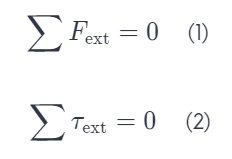The first condition is the condition for translational equilibrium, and the second is the condition for rotational equilibrium.

### Given Data

The length of the board is: L=3.00 m.

The board is uniform.

The weight of the board is: w=160 N.

The force applied by one person at one end of the board is: F_1=60 N.

### Required Data

We are asked to determine the location of the point at which the other person lifts.

### Solution

Since the board is uniform, its center of gravity is located at its geometrical center. The free-body diagram shown below shows the forces exerted on the board; F1 is the force applied by one person at one end of the board, F2 is the force applied by the other person, and 160 N is the gravitational weight of the board.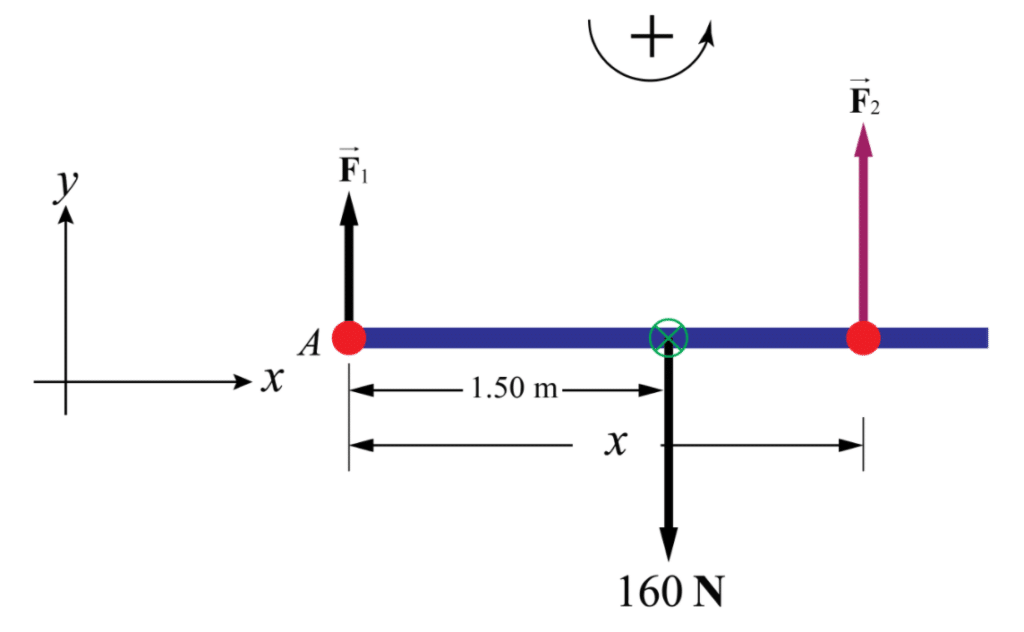Let upwards be positive. Apply the first condition for translational equilibrium from Equation (1) to the board: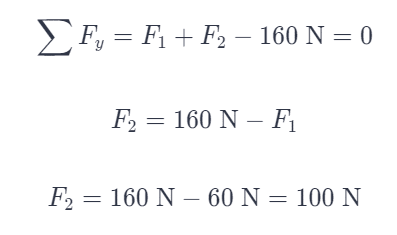Let counterclockwise rotations be positive. Apply the second condition for rotational equilibrium from Equation (2) about an axis of rotation through point A: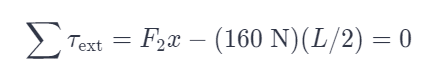where x is the moment arm of F2 and L/2 is the moment arm of the gravitational force. Rearrange and solve for x: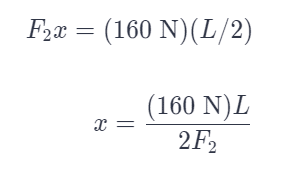Substitute numerical values: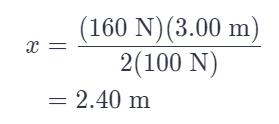Therefore, the other person should lift with a force of 100 N a distance 2.40 m from the end of the board.